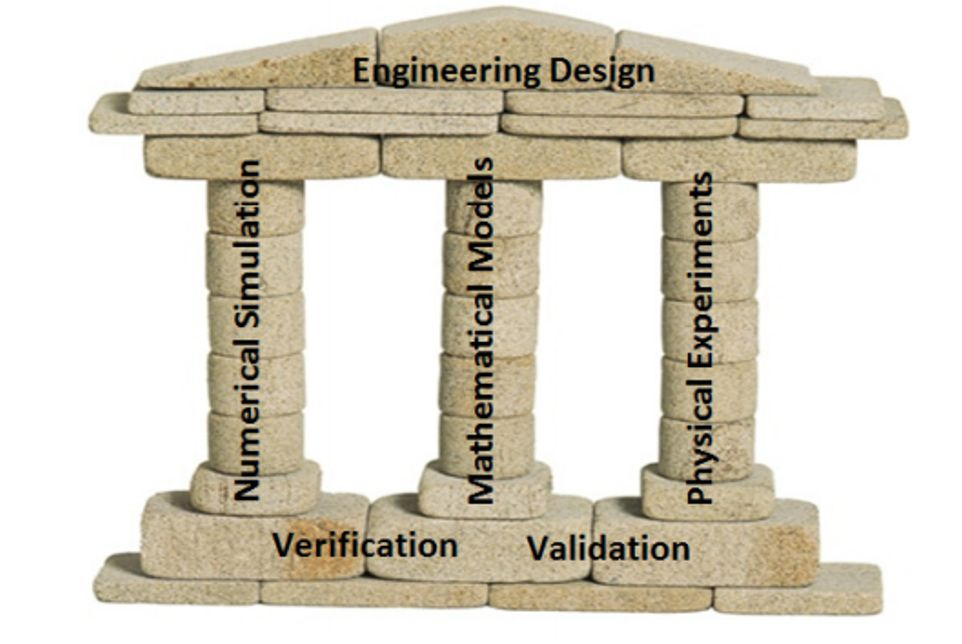# Simulation Governance

## Introduction

Simulation governance is a term coined by Professor Barna Szabo in an article written for Digital Engineering in 2015 - A Case for Simulation Governance. The following figure illustrates the supports of good engineering design and how verification and validation are essential. Once verification and validation have been achieved then it is possible to use numerical simulation to model physical reality.

In his Keynote Presentation at NAFEMS World Congress 2017 (Stockholm), Barna defined Simulation Governance as:

What it takes for organisations to develop confidence in the results of their numerical simulation projects.### Verification

Verification is the process whereby numerical simulation, e.g., finite element analysis, is matched to the underlying mathematical model.

### Validation

Validation is the process whereby physical experiments are matched to the underlying mathematical model.

### Benchmark Problems

Benchmark problems provide theoretical solutions to a problem of interest so that software verification can be undertaken. In some cases, where theoretical solutions are not available, numerical solutions of sound provenance are adopted.